Courses

# Past Year Questions: Linear Programming

## 20 Questions MCQ Test Engineering Mechanics | Past Year Questions: Linear Programming

Description
This mock test of Past Year Questions: Linear Programming for Mechanical Engineering helps you for every Mechanical Engineering entrance exam. This contains 20 Multiple Choice Questions for Mechanical Engineering Past Year Questions: Linear Programming (mcq) to study with solutions a complete question bank. The solved questions answers in this Past Year Questions: Linear Programming quiz give you a good mix of easy questions and tough questions. Mechanical Engineering students definitely take this Past Year Questions: Linear Programming exercise for a better result in the exam. You can find other Past Year Questions: Linear Programming extra questions, long questions & short questions for Mechanical Engineering on EduRev as well by searching above.
QUESTION: 1

### In an assembly line for assembling toys, five workers are assigned tasks which take times of 10, 8, 6, 9 and 10 minutes respectively. The balance delay for line is 

Solution:

Assuming cycle time = 10 + 8 + 6 + 9 + 10 = 43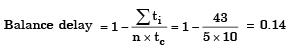= 14%

QUESTION: 2

Solution:
QUESTION: 3

### A manufacturer produces two types of products, 1 and 2, at production levels of x1 and x2 respectively. The profit is given is 2x1 + 5x2. The production constraints are: x1 + 3x2 < 40 3x1 + x2 < 24 x1 + x2 < 10 x1 > 0 x2 > 0 The maximum profit which can meet the constraints is 

Solution: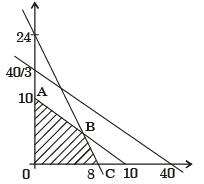Feasible Region (0– A – B – C– 0) At point (A) 0, 10) Z = 2 (0) + 5 (10) = 50
At point B (7,3) Z = 2(7) + 5(3) = 2y At point C (8, 0) Z = 2(16) + 5(0) = (16) 2
⇒32
Hence maximum profit is Zmax = 50
No correct option is given.

QUESTION: 4

A component can be produced by any of the four processes, I, II, III and IV. Process I has fixed cost of Rs. 20 and variable cost of Rs. 3 per piece.Process II has a fixed cost of Rs. 50 and variable cost of Rs. 1 per piece. Process III has a fixed cost of Rs. 40.00 and variable cost of Rs. 2 per piece. Process IV has fixed cost of Rs. 10 and Variable cost Rs. 4 per piece. If company wishes to produce 100 pieces of the component, from economic point of view it should choose

[2005 : 2 Marks]

Solution: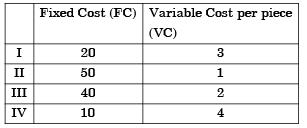Total cost = Fixed Cost (FC) + Number of piece (n) × Variable Cost per piece (VC) TC = FC + (n) x V.C
For I
TCI = 20+ (100) 3 = 320
For II
TCII = 50 + (100) 1 = 150
For III
TCIII = 40 + 100 (2) = 240
ForIV
TCIV = 10 + 100 (4) = 410
So from economical point of view, one should chose process II

QUESTION: 5

A company has two factories S1, S2 and two warehouses D1, D2. The supplies from S1 and S2 are 50 and 40 units respectively. Warehouse D1 requires a minimum of 20 units and a maximum of 40 units. Warehouse D2 requires a minimum of 20 units and, over and above, it can take as much as can be supplied. A balanced transportation problem is to be formulated for the above situation. The number of supply points, the number of demand points, and the total supply (or total demand) in the balanced transportation problem respectively are



Solution: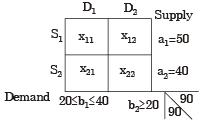Total no of supply point
⇒ m + n – 1
⇒ 2 + 2 – 1
⇒ 3
Total no of Demand point = 4
(x11, x12, x21, x22)
Total supply = Total Demand Þ 90 units

QUESTION: 6

If an additional constraint X1 + X2 < 5 is added, the optimal solution is



Solution:

Max z = x1 + x2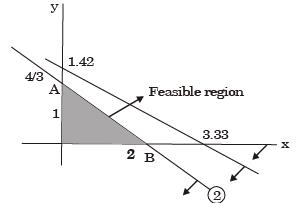As feasibly region remains the same solution remains the same (4/3, 4/3).
Hence, the correct option is (b).

QUESTION: 7

Let Y1 and Y2 be the decision variables of the dual and v1 and v2 be the slack variables of the dual of the given linear programming problem.The optimum dual variables are



Solution:

The optimal dual variables are V1 & V2 .

QUESTION: 8

A firm is required to procure three items (P, Q, and R). The prices quoted for these items (in Rs.) by suppliers S1,  S2 and S3 are given in table. The management policy requires that each item has to be supplied by only one supplier and one supplier supply only one item.The minimum total cost (in Rs.) of procurement to the firm is



Solution:
QUESTION: 9

Consider the following Linear Programming Problem (LPP):
Maximize z = 3x1 + 2x2,
Subject to x1 < 4 x2 < = 6
3x1 + 2x2 < 18
x1 > 0, x2 > 0



Solution:

z= 3 × 1 + 2 × 2
x1 ≤ 4
x2 ≤ 6
3x1 + 2x≤ 18
x1,x2 ≥ 0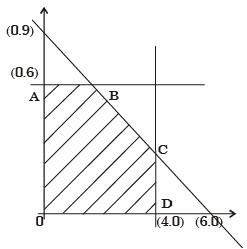QUESTION: 10

One unit of product P1 requires 3 kg of resource R1 and 1 kg resource R2. One unit of product P2 requires 2 kg of resource R1 and 2 kg of resource R2. The profits per unit by selling product P1 and P2 and Rs. 12000 and Rs 3000 respectively. The manufacturer has 90 kg of resource R1, and 100 kg of resource R2.

The unit worth of resource R2, i.e. dual price of resource R2 in Rs per kg is



Solution:

Because second constraint is redundant in nature. Therefore, resource R2 has no effect on the feasible solution.

QUESTION: 11

The manufacturer can make a maximum profit of  Rs.



Solution:

z = 2000 P1 + 3000 P2
Subjected to
3P1 +2P2 ≤ 90
P1 + 2P2 ≤ 100
P1, P2 ≥ 0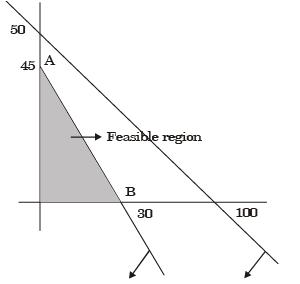At point A (30, 0) Z = 30 × 2000 × 3000 × 0 = 6000
At point B (0, 45) Z = 2000× 0 + 3000 × 45 = 135000
Hence Maximum Profit
[Zmax = 135000]

QUESTION: 12

A linear programming problem is shown below:
Maximise 3x + 7y
Subjeot to 3x + 7y < 10
4x + 6y < 8 x, y > 0



Solution:

z = 3x + 7y Constraints 3x + 7y ≤ 10 4x + 6y < 8; x, y ≥ 0 Corresponding equations 3x + 7y = 10; 4x + 6y =8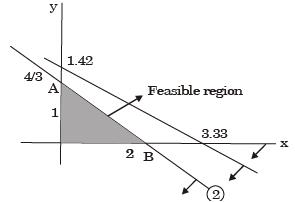A  (0, 4/3) z = 9.23 B (2, 0) z = 6 Thus, exactly one optimal solution.
Hence, the correct option is (b).

QUESTION: 13

Maximize z = 15x1 + 20x2
Subject to 12x1 + 4x2 >  36
12x1 + 6x2 > 24 x1,
x2 > 0
The above linear programming problem has

Solution:

zmax = 16x1 + 20x2
12x1 + 4x2 ≥36
12x1 + 6x2 ≥24 x1
x2 ≥ 0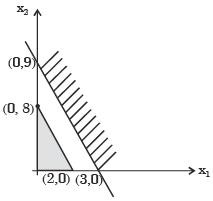QUESTION: 14

For the linear programming problem:
Maximize z = 3x1 + 2x2
Subject to –2x1 + 3x2 < 9x1 – 5x2 > – 20x1, x2 > 0
The above problem has



Solution:

Maximize Z= 3X+ 2X2
Subject to
-2X+ 3X≤ 9
X1 - 5X≥ 20
XP X2 ≥ 0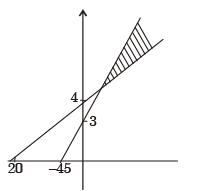The LPP is  unbounded.

QUESTION: 15

For the standard transportation linear program with m sources and n destinations and total supply equaling total demand, an optimal solution (lowest cost) with the smallest number of non-zero xij values (amounts from source i to destination j) is desired. The best upper bound for this number is



Solution:

In such an L .P.P, m × n variables are th ere an d m + n equations/constraints are there (satisfying the demand-supply requirements). But one constraint is removed as total supply equals total demand.  The best upper bound xij values is (m + n  1).

QUESTION: 16

After introducing slack variables s and t, the initial basic feasible solution is represented by the table below (basic variables are s = 6 and t = 6, and the objective function value is 0).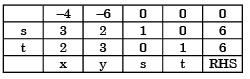After some simplex iterations, the following table is obtained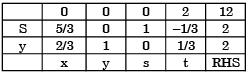From this, one can conclude that



Solution:

z = 4x + 6y
3x + 2y ≤ 6
2x + 3y ≤ 6
x, y > 0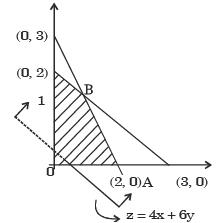Feasible region (O–A–B–C–0) Since, Slope of objective function is equal to the slope of constraint Hence LPP has multiple optimal solution
At B (6/5, 6/5)
Z = 12
At C (0,2)
Z = 12

QUESTION: 17

The dual for the LP in Q. 21 is



Solution:
QUESTION: 18

Simplex method of solving linear programming problem uses



Solution:
QUESTION: 19

A company produces two types of toys : P and Q. Production time of Q is twice that of P and the company has a maximum of 2000 time units per day. The supply of raw material is just sufficient to produce 1500 toys (of any type) per day. Toy type Q requires an electric switch which is available @ 600 pieces per day only.The company makes a profit of Rs. 3 and Rs. 5 on type P and Q respectively. For maximization​of profits, the daily production quantities of P and Q toys should respectively be



Solution:

Zmax = 3P +5Q
subject to
P+ 2Q ≤ 2000
P+Q ≤ 1500
Q ≤ 600
P,Q ≥ 0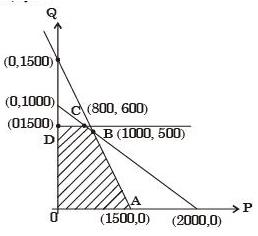Feasible solution (O A B C D) Since At point A (1500,0) Z = 3 × 1500 + 5 × 0 = 4500
At Point B (1000, 500) Z = 3 × 1000 + 5 × 500 = 5500
At point C (800, 600) Z = 3 × 800 + 5 × 600 = 5400
At Point O (0, 600) Z = 3 × 0 + 5 × 600 = 3000
Hence Z in maximum at B (1000,500) P = 1000 units Q = 500 units

QUESTION: 20

The manufacturer can make a maximum profit of  Rs.



Solution: